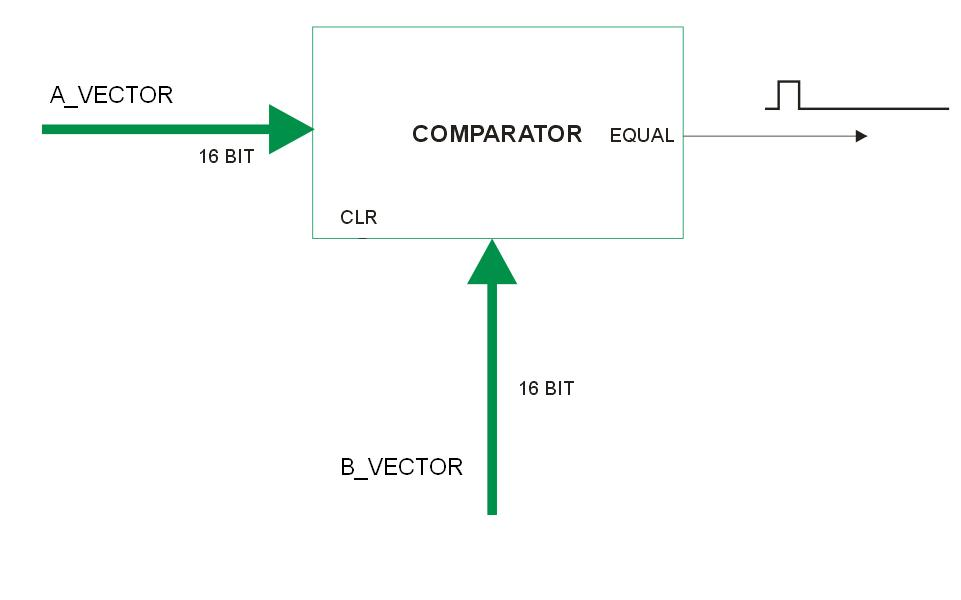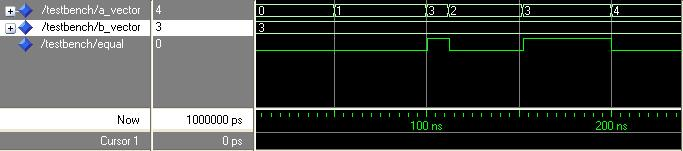# FVBE - EqualComparator16bit1

by Roberto Asquini

#### Make a simple equality comparator with 16 bitBlock diagram of the EqualComparator16bit1 VHDL code

This example presents another very simple peripheral written in VHDL. We will show the VHDL code and the results of its simulation with the Libero suite. For a working example using this comparator as a building block please refer to the Fox VHDL by Example: EventCounter1 The source code for the 16 bit equal comparator we are presenting here is: EqualComparator16bit1.vhd.

The first lines are the declaration of the needed libraries:

```
library ieee;
use ieee.std_logic_1164.all;
use ieee.std_logic_unsigned.all;
library proasic3;
```
Then there is the entity declaration for this file that represents the interface port for this VHDL unit. Every other external block of VHDL code, to be able to use this counter, needs to declare the very same port interface as a component and needs to instantiate it.

This is the entity declaration:

```entity EqualComparator16bit1 is
port(
A_VECTOR : in std_logic_vector(15 downto 0); -- 16 bit vector A for the comparison.
B_VECTOR : in std_logic_vector(15 downto 0); -- 16 bit vector B for the comparison.
EQUAL : out std_logic -- positive equality output signal.
);
end EqualComparator16bit1;
```

In this entity we see the declaration of two 16 bit ports in input: A_VECTOR and B_VECTOR that receive the external 16 bit values to compare. The third port signal is an output: EQUAL that will become high whenever the two vectors A and B are equal.

Now, continuing in the file, there is the architecture declaration. It is called Dataflow since the only statement realizing the complete equality comparator is a direct statement. Direct statements (not inside a process) are used in the concurrent way to describe a logic circuit since they are all executed in parallel. The concurrent way (it means the parallel execution way) is called also Dataflow:

```
architecture Dataflow of EqualComparator16bit1 is

begin

EQUAL <= '1' when A_VECTOR = B_VECTOR else '0';

end Dataflow;
```

No processes are needed here since the equal comparator is a pure combinatorial function.

The single line between begin and end is self explaining. The EQUAL port signal is put at '1' logic state when the two input vectors are equal. Otherwise the EQUAL port signal is maintained at '0' level.

From this little example you can see the concise and clear way to express statements that will involve lots of logic gates. The power of expression of VHDL can be seen if we would like to change the design up to a 128 bit comparator for example. We will have changed only the bounds inside the entity declaration but no change will be needed inside the architecture declaration since the logic operation is the same.

### Simulation of EqualComparator16bit1.vhd

In the following you can see the simulation output diagram of the EqualComparator16bit1.vhd VHDL code. This simulation has been realized using the standard tools inside the Actel suite of programs Libero 7.1:Simulation diagram of EqualComparator16bit1 VHDL code

Remember that VHDL is not case sensitive! So, even if we declared the port signals in upper case, the simulator shows anyway all names in lower case.

It is possible to see that immediately after the two 16 bit vectors become equal the output equal is set to high logic level.

This example is very simple but is completely working. If we should map the A_VECTOR, the B_Vector and the EQUAL output on physical external pins of the FOX_VHDL FPGA, we could have the comparator hardware function up and running. More interesting it will surely be instead to use this function inside some other more interesting peripheral as a building block like in this example: Fox VHDL by Example: EventCounter1.